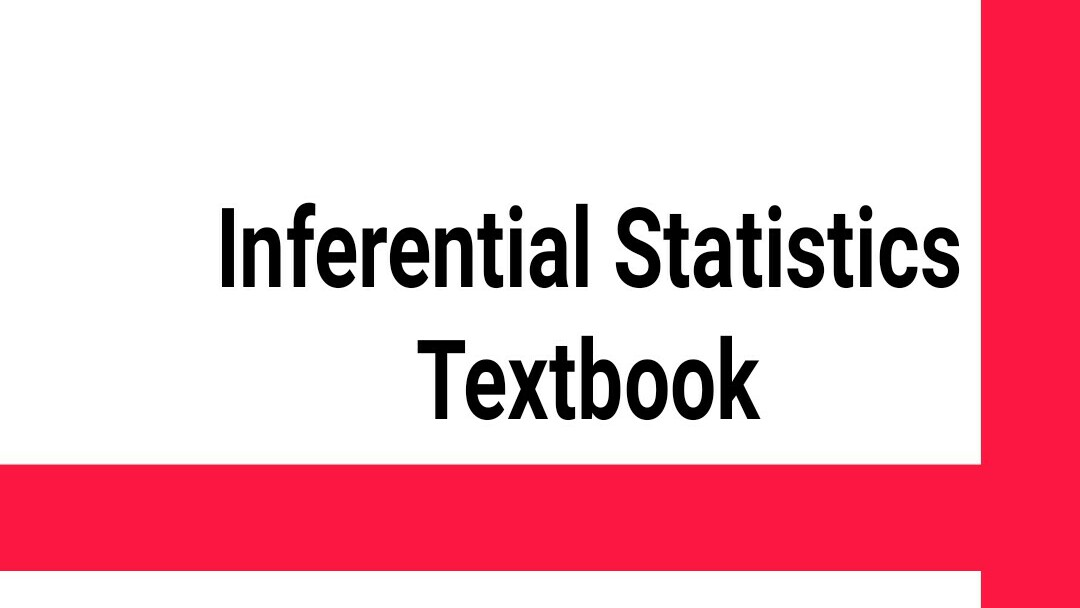-->Making a reasonable decision and drawing a valid conclusion about the sample drawn from the population is what referred to as statistics based on my experience. Its good enough to every statistician to be able to understand and masters every unit in statistical inferential statistics, with due to that haskenews.com.ng is capable to introduce you best and understandable statistical inferential statistics textbook for undergraduates and postgraduates students. Note this textbook is special to to those who want to undergoes their knowledge and further their exBinomial Theorem.

Hypothesis Testing.

Experimental Analysis

Normal Distributions.

T-Distributions.

Central Limit Theorem.

Confidence Intervals.

Regression Analysis / Linear Regression.

Comparison of Means.

perience in statistics. Some of the topics you may found in this textbook include: